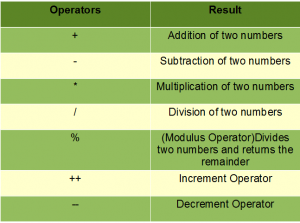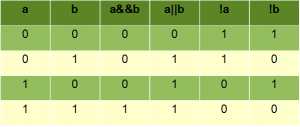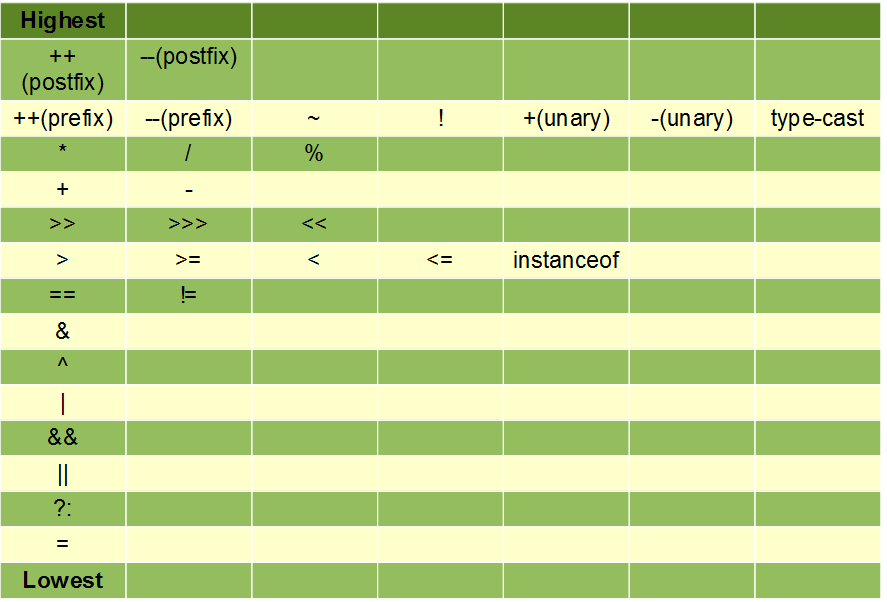# Basic Operators in Java

Java provides a rich operator environment. We can classify the basic operators in java in the following groups:

• Arithmetic Operators
• Relational Operators
• Bitwise Operators
• Assignment Operators
• Logical Operators

Let us now learn about each of these operators in details.

1. Arithmetic Operators: Arithmetic operators are used to perform arithmetic/mathematical operations on operands.
• Subtraction (‘-‘):  Subtracts two operands
• Multiplication (‘*’):  Multiplies two operands
• Division (‘/’): Divides the first operand by the second.
• Modulus (‘%’): Returns the remainder when first operand is divided by the second
• Increment (‘++’): Increment the value of an integer. When placed before the variable name (also called pre-increment operator), its value is incremented instantly. For example, ++x.And when it is placed after the variable name (also called post-increment operator), its value is preserved temporarily until the execution of this statement and it gets updated before the execution of the next statement. For example, x++.
• Decrement (‘–‘): Decrement the value of an integer. When placed before the variable name (also called pre-decrement operator), its value is decremented instantly. For example, –x.
And when it is placed after the variable name (also called post-decrement operator), its value is preserved temporarily until the execution of this statement and it gets updated before the execution of the next statement. For example, x–.

Note: The increment and decrement operators are called unary arithmetic operators as they work with a single operand whereas the rest of arithmetic operators are called binary arithmetic operators as they operate on two operands.`//Java program to explain arithmetic operators ` `import` `java.util.*; ` `class` `A{ ` `     `  `    ``public` `static` `void` `main(String args[]) ` `    ``{ ` `     `  `    ``int` `a = ``10``, b = ``4``, res; ` `  `  `    ``//printing a and b ` `    ``System.out.println(``"a is "``+a+ ``" and b is "``+ b); ` `  `  `    ``res = a+b; ``//addition ` `    ``System.out.println(``"a+b is "``+res); ` `  `  `    ``res = a-b; ``//subtraction ` `    ``System.out.println(``"a-b is "``+res); ` `  `  `    ``res = a*b; ``//multiplication ` `    ``System.out.println(``"a*b is "``+res); ` `  `  `    ``res = a/b; ``//division ` `    ``System.out.println(``"a/b is "``+res); ` `  `  `    ``res = a%b; ``//modulus ` `    ``System.out.println(``"a%b is "``+res); ` `     `  `    ``} ` `} `

Output:

```a is 10 and b is 4
a+b is 14
a-b is 6
a*b is 40
a/b is 2
a%b is 2
```
2. Relational Operators: The relational operators determine the relationship that one operand has to the other. The relational operators evaluates the relation between two operations and returns true if the relation exists else false.
• ‘==’ operator:  checks whether the two given operands are equal or not. If so, it returns true. Otherwise it returns false. For example, 5==5 will return true.
• ‘!=’ operator:  checks whether the two given operands are equal or not. If not, it returns true. Otherwise it returns false. It is the exact boolean complement of the ‘==’ operator. For example, 5!=5 will return false.
• ‘>’ operator: checks whether the first operand is greater than the second operand. If so, it returns true. Otherwise it returns false. For example, 6>5 will return true.
• ‘<‘ operator:checks whether the first operand is lesser than the second operand. If so, it returns true. Otherwise it returns false. For example, 6<5 will return false.
• ‘>=’ operator: checks whether the first operand is greater than or equal to the second operand. If so, it returns true. Otherwise it returns false. For example, 5>=5 will return true.
• ‘<=’ operator: checks whether the first operand is lesser than or equal to the second operand. If so, it returns true. Otherwise it returns false. For example, 5<=5 will also return true.

 `//Java program for relational operators ` `import` `java.util.*; ` `class` `A{ ` `     `  `    ``public` `static` `void` `main(String args[]) ` `    ``{ ` `     `  `    ``int` `a=``10``, b=``4``; ` `  `  `    ``// relational operators ` `    ``// greater than example ` `    ``if` `(a > b) ` `        ``System.out.println(``"a is greater than b"``); ` `    ``else` `System.out.println(``"a is less than or equal to b"``); ` `  `  `    ``// greater than equal to ` `    ``if` `(a >= b) ` `        ``System.out.println(``"a is greater than or equal to b"``); ` `    ``else` `System.out.println(``"a is lesser than b"``); ` `  `  `    ``// less than example ` `    ``if` `(a < b) ` `        ``System.out.println(``"a is less than b"``); ` `    ``else` `System.out.println(``"a is greater than or equal to b"``); ` `  `  `    ``// lesser than equal to ` `    ``if` `(a <= b) ` `        ``System.out.println(``"a is lesser than or equal to b"``); ` `    ``else` `System.out.println(``"a is greater than b"``); ` `  `  `    ``// equal to ` `    ``if` `(a == b) ` `        ``System.out.println(``"a is equal to b"``); ` `    ``else` `System.out.println(``"a and b are not equal"``); ` `  `  `    ``// not equal to ` `    ``if` `(a != b) ` `        ``System.out.println(``"a is not equal to b"``); ` `    ``else` `System.out.println(``"a is equal b"``); ` `  `  `         `  `    ``} ` `} `

Output:

```a is greater than b
a is greater than or equal to b
a is greater than or equal to b
a is greater than b
a and b are not equal
a is not equal to b
```
3. Bitwise Operators: Java provides several bitwise operators to work with integer types , long , int , short , char , byte. Bitwise operators performs bit by bit operation on binary representation of integers. These operators acts upon he individual bits of their operands.
For Example –

```Assume a = 9 and b = 7.
In binary form,
a = 1001
b = 0111
----------
a & b = 0001
```

Different bitwise operators available in Java are:

• & (bitwise and): Bitwise & operator performs binary AND operation bit by bit on the operands. a&b = 0001 which is 1
• | (bitwise or): Bitwise | operator performs binary OR operation bit by bit on the operands. a|b = 1111 which is 15
• ^ (bitwise XOR): Bitwise ^ operator performs binary XOR operation bit by bit on the operands. a^b = 1110 which is 14
• ~ (bitwise compliment):Bitwise ~ operator performs binary NOT operation bit by bit on the operand. ~b = 1000 which is 8
• << (left shift): This operator shifts the bits of the left operand to left by number of times specified by right operand. a<<1 = 10010 = 18
• >> (right shift): This operator shifts the bits of the left operand to right by number of times specified by right operand. a>>1 = 0100 = 4
• >>> (zero fill right shift): Shift right zero fill operator.The left operand value is shifted to right by number of digits specified by right operand and shifted digits will be replaced by zero. a>>>2 = 0010 = 2
4. Assignment Operator: The assignment operator is used to assign value to a variable. The general form of an assignment operator is:

var = expression

Different ways of using assignment operator:

• ‘=’: This is the simplest assignment operator. It assigns the value of left operand to the right operand. For example, a = 3.
• ‘+=’: This operator first adds the left and right operands and then assigns the result to the left operand. For example, a += b is equivalent to a = a + b.
• ‘-=’: This operator first subtracts the right operand from left operand and then assign the result to left operand. For example, a -= b is equivalent to a = a – b.
• ‘*=’: This operator first multiplies the right operand and left operand and then assign the result to left operand. For example, a *= b is equivalent to a = a * b
• ‘/=’: This operator first divides the left operand by right operand and then assign the result to left operand. For example, a /= b is equivalent to a = a / b
• ‘%=’: This operator calculates modulus using left and right operand and then assigns the result to the left operand. For example, a %= b is equivalent to a = a % b

Similarly we can also use operators like , ^= , &= , |=.

5. Logical Operators: Logical operators perform logical operations like logical AND, logical OR etc. Let us assume that variable a holds the boolean value true and b holds the boolean value false. Below are some logical operators we can use:
• Logical AND (‘&&’): This operator will return true if both the left and right operands are true, otherwise it will return false. For example, a && b is false.
• Logical OR (‘||’): This operator will return true if any one of the left and right operands are true. It will return false when both left and right operands are false. For example, a || b is True.
• Logical NOT (‘!’): This is a unary operator and can be used with a single operand. This will return true if the operand is false and return false if the operand is true. For example, !a is false and !b is true.

Sample truth table with two variables:6. Other Operators
• instanceof Operator:
As the name suggests this operator is to check whether a object or reference variable is of particular class type or interface type or not. Syntax to use instanceof operator:

```(Object or reference variable ) instanceof  (class or interface type)
```

This operator return a boolean value either true or false. If the object on the left side of the operator is of the type of class on the right side of the operator then the operator will true otherwise false.

 `//Java program for instanceof operator ` ` `  `public` `class` `InstanceOf { ` ` `  `   ``public` `static` `void` `main(String args[]) { ` ` `  `      ``String name = ``"GeeksforGeeks"``; ` `         `  `      ``//instanceof operator will return true here  ` `      ``//as the object name is of type String ` `      ``boolean` `res = name ``instanceof` `String; ` `      ``System.out.println( res ); ` `   ``} ` `} `

Output:

```true
```
• conditional Operator: We normally use the if-then-else statements fr evaluating conditions. Java include a special operator ‘?’ which can replace if-then-else statements for evaluating conditions. The general form of ‘?’ operator is:
```expression1 ? expression2 : expression3

expression1: expression that evaluates to a boolean value i.e. either true or false.
expression2: if expression1 evaluates to true then expression2 is evaluated
expression3: if expression1 evaluates to false then expression3 is evaluated.
```

 `//Java program to illustrate coditional operators ` ` `  `class` `Conditional ` `{ ` `    ``public` `static` `void` `main(String args[]) ` `    ``{ ` `        ``int` `num1 = ``4``; ` `        ``int` `num2 = ``5``; ` `         `  `        ``//using conditional operator ` `        ``num1 = num1>num2 ? num1 - num2 : num1 + num2; ` `         `  `        ``//num1 stores the value after evaluation of either second  ` `        ``// or third expression depending on the condition  ` `        ``//provided in the first expression ` `        ``//num1 becomes 4+5 = 9 ` `         `  `        ``//printing num1 ` `        ``System.out.println(num1); ` `         `  `    ``} ` `} `

Output:

`9`

Precedence Chart

Below table shows the precedence order of operators from highest to lowest. Operators in same row have equal precedence.This article is contributed by Harsh Agarwal. If you like GeeksforGeeks and would like to contribute, you can also write an article using contribute.geeksforgeeks.org or mail your article to contribute@geeksforgeeks.org. See your article appearing on the GeeksforGeeks main page and help other Geeks.